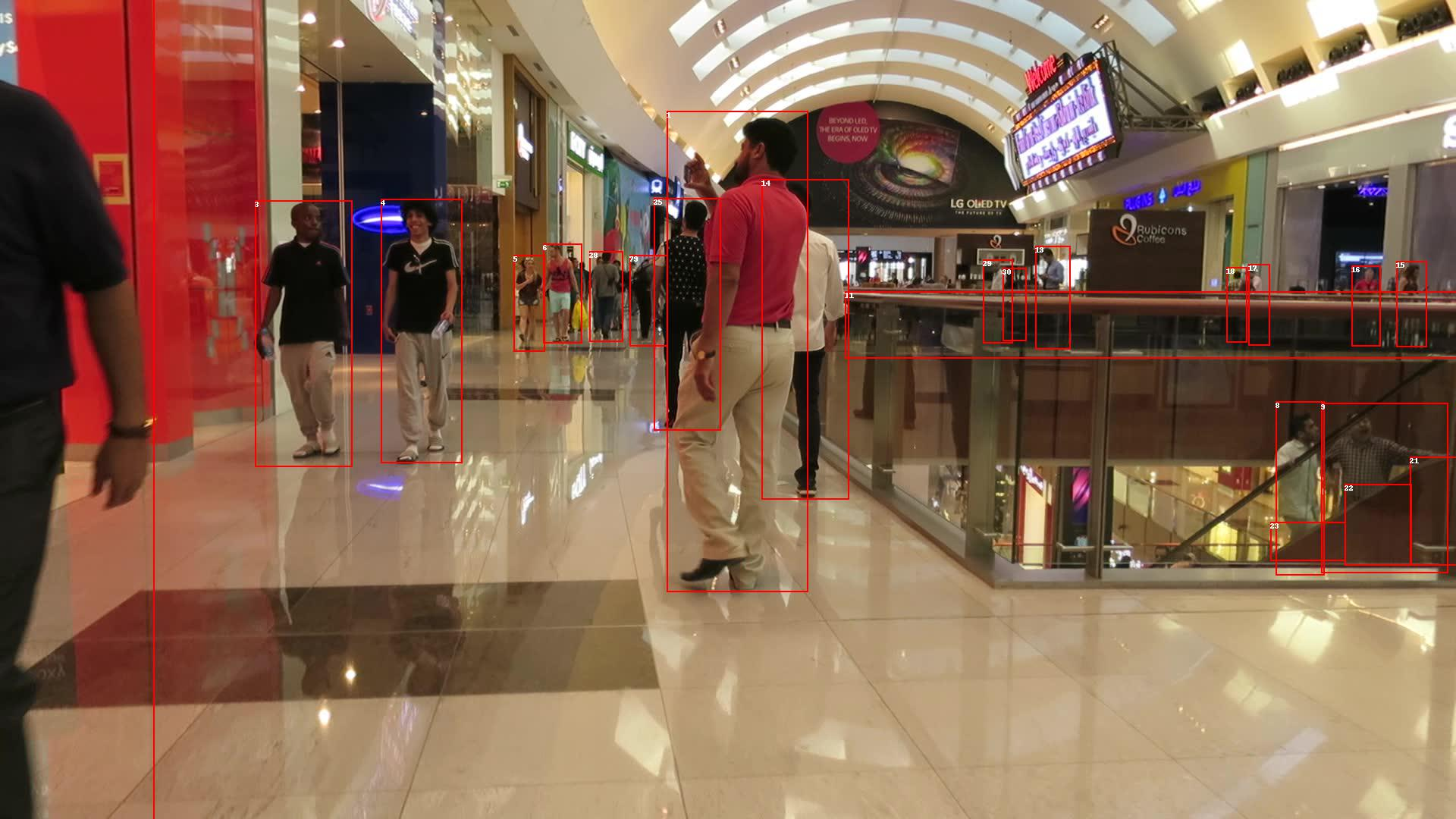Comment on page

# Creating Datasets with Sequences

Deep Lake sequences are a powerful tool for storing temporal annotations such as bounding boxes in each frame of a video.

## How to create a dataset with sequences of images and labels

#### This tutorial is also available as a Colab Notebook​

Deep learning with computer vision is increasingly moving in a direction of temporal data, where video frames and their labels are stored as sequences, rather than independent images. Models trained on this data directly account for the temporal information content, rather than making predictions frame-by-frame and then fusing them with non-deep-learning techniques.

### Create the Deep Lake Dataset

The dataset has the following folder structure:
data_dir
|_train
|_MOT16_N (Folder with sequence N)
|_det
|_gt (Folder with ground truth annotations)
|_img1 (Folder with images the sequence)
|_00000n.jpg (image of n-th frame in sequence)
|_MOT16_M
....
|_test (same structure as _train)
The annotations in `gt.txt` have the format below, and the last 4 items (conf->z) are not used in the Deep Lake dataset:
frame, id, bb_left, bb_top, bb_width, bb_height, conf, x, y, z
Now we're ready to create a Deep Lake Dataset in the `./mot_2016_train` folder by running:
import deeplake
import os
import pandas as pd
import numpy as np
from PIL import Image, ImageDraw
ds = deeplake.empty('./mot_2015_train') # Create the dataset locally
Next, let's write code to inspect the folder structure for the downloaded dataset and create a list of folders containing the sequences:
dataset_folder = '/MOT16/train'
sequences = [ item for item in os.listdir(dataset_folder) if os.path.isdir(os.path.join(dataset_folder, item)) ]
Finally, let's create the tensors by using the `sequence[...]` htype, iterate through each sequence, and iterate through each frame within the sequence, one-by-one.
Data is appended to `sequence[...]` htypes using lists. The list contains the whole sample, and the individual elements of the list are the individual data points, such as the image frame, the bounding boxes in a particular frame, etc.
See end of code block below.
with ds:
# Define tensors
ds.create_tensor('frames', htype = 'sequence[image]', sample_compression = 'jpg')
ds.create_tensor('boxes', htype = 'sequence[bbox]')
ds.create_tensor('ids', htype = 'sequence[]', dtype = 'uint32') # Ids are not uploaded as htype = 'class_labels' because they don't contain information about the class of an object.
ds.boxes.info.update(coords = {'type': 'pixel', 'mode': 'LTWH'}) # Bounding box format is left, top, width, height
# Iterate through each sequence
for sequence in sequences:
# Define root directory for that sequence
root_local = os.path.join(dataset_folder,sequence, 'img1')
# Get a list of all the image paths
img_paths = [os.path.join(root_local, item) for item in sorted(os.listdir(root_local))]
# Read the annotations and convert to dataframe
with open(os.path.join(dataset_folder,sequence, 'gt', 'gt.txt')) as f:
anns = [line.rstrip('\n') for line in f]
# Get the frames from the annotations and make sure they're of equal length as the images
frames = pd.unique(anns_df)
assert len(frames) == len(img_paths)
# Iterate through each frame and add data to sequence
boxes_seq = []
ids_seq = []
for frame in frames:
ann_df = anns_df[anns_df == frame] # Find annotations in the specific frame
boxes_seq.append(ann_df.loc[:, [2, 3, 4, 5]].to_numpy().astype('float32')) # Box coordinates are in the 3rd-6th column
ids_seq.append(ann_df.loc[:, 1].to_numpy().astype('uint32')) # ids are in the second column
# Append the sequences to the deeplake dataset
ds.append({
"frames": [deeplake.read(path) for path in img_paths],
"boxes": boxes_seq,
"ids": ids_seq})
This dataset identifies objects by `id`, where each `id` represents an instance of an object. However, the `id` does not identify the class of the object, such `person`, `car`, `truck`, etc. Therefore, the `ids` were not uploaded as `htype = "class_label"`.

### Inspect the Deep Lake Dataset

Let's check out the 10th frame in the 6th sequence in this dataset. A complete visualization of this dataset is available in Activeloop Platform.
# Draw bounding boxes for the 10th frame in the 6th sequence
seq_ind = 5
frame_ind = 9
img = Image.fromarray(ds.frames[seq_ind][frame_ind].numpy())
draw = ImageDraw.Draw(img)
(w,h) = img.size
boxes = ds.boxes[seq_ind][frame_ind].numpy()
for b in range(boxes.shape):
(x1,y1) = (int(boxes[b]), int(boxes[b]))
(x2,y2) = (int(boxes[b]+boxes[b]), int(boxes[b]+boxes[b]))
draw.rectangle([x1,y1,x2,y2], width=2, outline = 'red')
# Display the frame and its bounding boxes
imgCongrats! You just created a dataset using sequences! 🎉